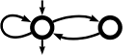Awali Another Weighted Automata library
abstract_context.hh
Go to the documentation of this file.
1 // This file is part of Awali.
2 // Copyright 2016-2021 Sylvain Lombardy, Victor Marsault, Jacques Sakarovitch
3 //
4 // Awali is a free software: you can redistribute it and/or modify
5 // it under the terms of the GNU General Public License as published by
6 // the Free Software Foundation, either version 3 of the License, or
7 // (at your option) any later version.
8 //
9 // This program is distributed in the hope that it will be useful,
10 // but WITHOUT ANY WARRANTY; without even the implied warranty of
11 // MERCHANTABILITY or FITNESS FOR A PARTICULAR PURPOSE. See the
12 // GNU General Public License for more details.
13 //
14 // You should have received a copy of the GNU General Public License
15 // along with this program. If not, see <http://www.gnu.org/licenses/>.
16
17 #ifndef DYN_ABSTRACT_CONTEXT_HH
18 #define DYN_ABSTRACT_CONTEXT_HH
19
20 #include<memory>
21 #include<string>
22 #include<vector>
23 #include<list>
24
25 #include <awali/dyn/core/any.hh>
28
29 namespace awali { namespace dyn {
30  class ratexp_t;
31  class automaton_t;
32
37
43  virtual std::string sname() const =0;
44
51  virtual std::string vname(bool full = true) const =0;
52
54  virtual std::string labelset_name() const =0;
55
57  virtual std::string weightset_name(std::string format="text") const =0;
58
60  virtual std::vector<any_t> alphabet() const = 0;
61
62  /* Returns the object representing epsilon.
63  *
64  * @beware Although printing the returned value of this function would give
65  * `\e`, it is not an std::string internally.
66  */
67  virtual label_t epsilon() const = 0;
68
70  virtual bool is_eps_allowed() const = 0;
71
75
77  virtual void add_letter(label_t let) = 0;
78
80  virtual bool has_letter(label_t let) const = 0;
81
84  virtual bool show_one() const = 0;
85
88  virtual weight_t weight_one() const = 0;
89
93  virtual weight_t weight_zero() const = 0;
94
96  virtual bool is_finite_weightset() const =0;
97
100  virtual bool is_locally_finite_weightset() const =0;
101
103  virtual weight_t add_weights(weight_t l, weight_t r) const = 0;
104
106  virtual weight_t mul_weights(weight_t l, weight_t r) const = 0;
107
109  virtual weight_t div_weights(weight_t l, weight_t r) const = 0;
110
112  virtual weight_t get_word(std::string str) const =0;
113
115  virtual weight_t get_weight(std::string str) const =0;
116
118  virtual label_t get_label(std::string str) const =0;
119
122  virtual label_t get_label(std::string str, size_t i) const =0;
123
125  virtual std::string weight_to_string(weight_t w) const=0;
126
128  virtual std::string label_to_string(label_t w) const=0;
129
130
138  virtual std::list<any_t> expand_label(label_t lab) const=0;
139
140
142  ratexp_t make_ratexp(std::string str, bool fixed_alphabet = true);
143
146
147
148  virtual ~abstract_context_t() {}
149
150  protected:
161  virtual abstract_context_t* copy() = 0;
162  };
163
164
165 }}//end of ns awali::dyn
166 #endif
An automaton_t is essentially a shared pointer to an abstract_automaton_t, but also contains static f...
Definition: automaton.hh:93
Main class for representing rational expresson at the dynamical layer.
Definition: ratexp.hh:66
std::shared_ptr< context_description_impl > context_description
Definition: description_types.hh:31
auto format(const ValueSet &vs, const typename ValueSet::value_t &v, Args &&... args) -> std::string
Format v via vs.print.
Definition: stream.hh:109
static const std::string full
Completely version of Awali as a std::string.
Definition: version.hh:40
Main namespace of Awali.
Definition: ato.hh:22
Abstract interface representing the weightset and labelset of an automaton, a rational expression or ...
Definition: abstract_context.hh:36
virtual bool show_one() const =0
Returns true if one (neutral multiplicative element of the weightset) should be printed in expression...
virtual bool has_letter(label_t let) const =0
Tests whether let is a valid letter for this context.
virtual label_t get_label(std::string str, size_t i) const =0
Converts string s to a label of the i-th tape (transducer only).
virtual void add_letter(label_t let)=0
Adds a letter in the alphabet.
ratexp_t make_ratexp(std::string str, bool fixed_alphabet=true)
Builds a ratexp_t from its string-representation.
virtual std::string label_to_string(label_t w) const =0
Computes the string-representation of given label.
virtual weight_t get_word(std::string str) const =0
Converts given string to a weight.
virtual std::string weightset_name(std::string format="text") const =0
Gives the name of the weightset.
virtual std::vector< any_t > alphabet() const =0
Returns the alphabet.
virtual abstract_context_t * copy()=0
Makes a copy of this abstract_context_t.
virtual std::list< any_t > expand_label(label_t lab) const =0
Expands a label into a list of labels, one label per tape (transducer only).
virtual label_t epsilon() const =0
virtual bool is_eps_allowed() const =0
Returns true if epsilon is allowed as label.
virtual context::context_description description() const =0
Returns the context::context_description corresponding to this abstract_context_t.
virtual bool is_finite_weightset() const =0
Returns true if the weightset contains a finite number of elements.
virtual weight_t get_weight(std::string str) const =0
Converts given string to a weight.
virtual label_t get_label(std::string str) const =0
Converts given string to a label.
virtual std::string labelset_name() const =0
Gives the name of the labelset.
virtual ~abstract_context_t()
Definition: abstract_context.hh:148
virtual std::string vname(bool full=true) const =0
Returns the runtime internal name of the context.
virtual bool is_locally_finite_weightset() const =0
Returns true if all finitely-generated sub-semiring of the weightset are all finite.
virtual weight_t div_weights(weight_t l, weight_t r) const =0
Returns the quotient of given weights, as computed by the weightset.
virtual std::string sname() const =0
Returns the compile-time internal name of the context.
virtual std::string weight_to_string(weight_t w) const =0
Computes the string-representation of given weight.
virtual weight_t weight_zero() const =0
Gives the value zero, that is, the neutral additive element of the weightset.
virtual weight_t add_weights(weight_t l, weight_t r) const =0
Returns the sum of given weights, as computed by the weightset.
virtual weight_t mul_weights(weight_t l, weight_t r) const =0
Returns the product of given weights, as computed by the weightset.
virtual weight_t weight_one() const =0
Returns the value one, that is, the neutral multiplicative element of the weightset.
automaton_t make_automaton()
Builds an empty automaton_t with this context.
Structure used to erase the type of labels/weights at the dyn layer.
Definition: any.hh:52